# C 430: Private-Key Encryption With Sodium (65 extra)

## What You Need

Any machine with Python 3.

## Purpose

To learn how to use the Sodium library for private-key encryption.

## Installing and Importing PyNaCl

In a Terminal window, execute these commands:
```python3 -m pip install pynacl python3 import nacl.secret import nacl.utils import nacl.pwhash dir(nacl.secret) dir(nacl.secret.SecretBox) nacl.secret.SecretBox.KEY_SIZE ```
The nacl.secret library contains an object named SecretBox, which has an attribute named KEY_SIZE with a value of 32, as shown below.

This is the private key and it is 32 bytes long (256 bits).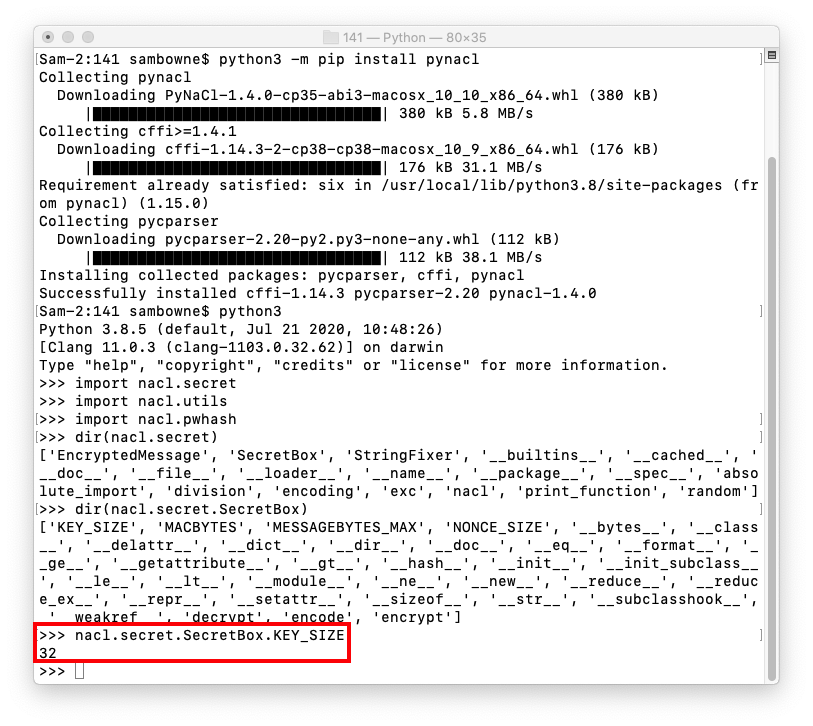## Using Help

To see more about Sodium and the the secret module, execute this command:
```help(nacl.secret) ```
You see a page with lots of information about the module, including the SecretBox class, as shown below.

To exit the help message, press Q.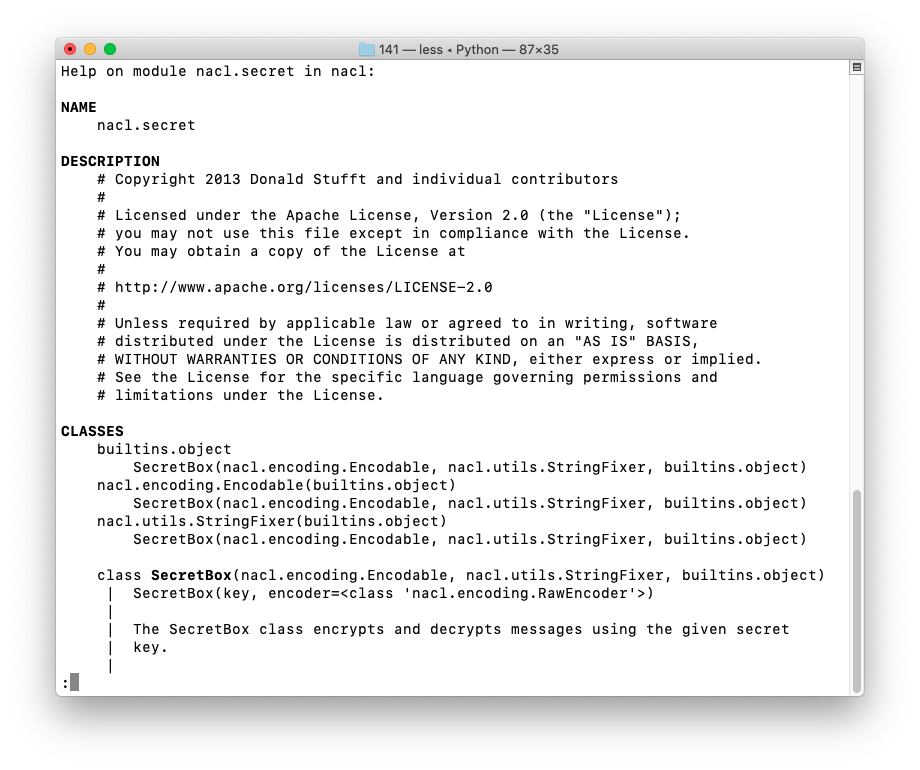## Understanding Key Derivation

We'll derive the key from a password using the Argon2 key derivation function. This was the winner of the Password Hashing Competition, so it's recognized as the best system to use by the world's experts in cryptography. If you want a complete explanation of it, see the Draft RFC.

To see how it works, execute these commands.

(Notice the definition of kdf -- that will make later commands shorter.)

```dir(nacl.pwhash) dir(nacl.pwhash.argon2i) dir(nacl.pwhash.argon2i.kdf) kdf = nacl.pwhash.argon2i.kdf help(kdf) ```
As shown below, the kdf function needs a password and a salt as inputs.

To exit the help message, press Q.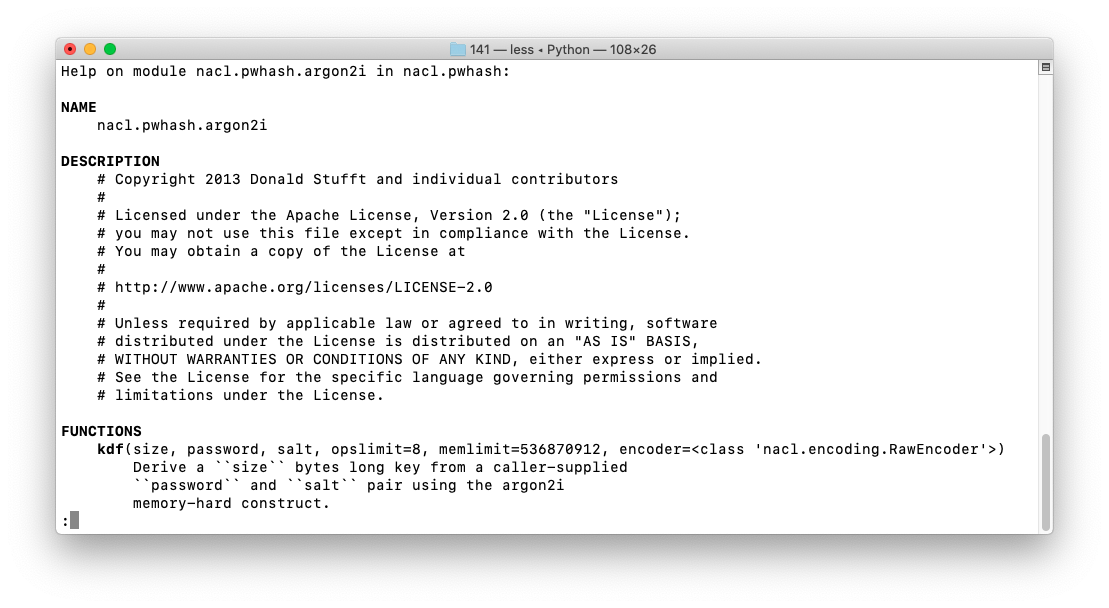## Performing Key Derivation

To generate a random salt, execute these commands:
```salt_size = nacl.pwhash.argon2i.SALTBYTES salt_size salt = nacl.utils.random(salt_size) salt ```
The salt size is 16 bytes. Now we have a random salt, as shown below.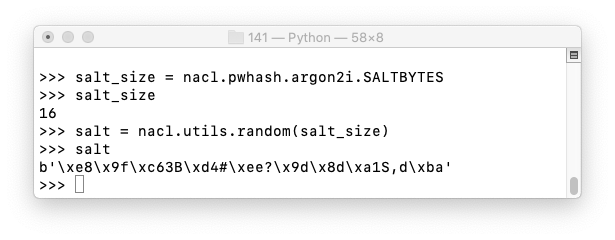To derive a key from the password topsecret, execute these commands:

```password = "topsecret".encode("utf-8") key = kdf(nacl.secret.SecretBox.KEY_SIZE, password, salt) key ```
This generates a key, as shown below.

The key derivation is slow--it took about 20 seconds on my system. This makes it very secure, because an attacker would need a very long time to generate a dictionary of password hashes.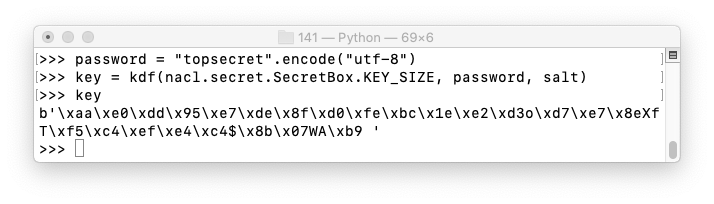The process is deterministic: if you save the the salt and the password, you can recalculate the private key from them.

## Encrypting a Plaintext Message

To encrypt a message saying HELLO, execute these commands:
```plaintext = b"HELLO" box = nacl.secret.SecretBox(key) ciphertext = box.encrypt(plaintext) ciphertext ```
This produces ciphertext containing random-looking bytes, as shown below.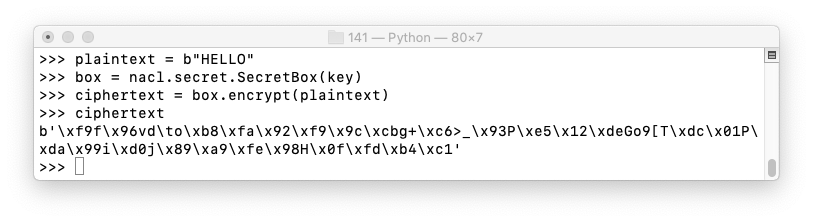## Decrypting the Ciphertext

To decrypt the ciphertext, execute these commands:
```decrypted = box.decrypt(ciphertext) decrypted ```
This produces ciphertext containing random-looking bytes, as shown below.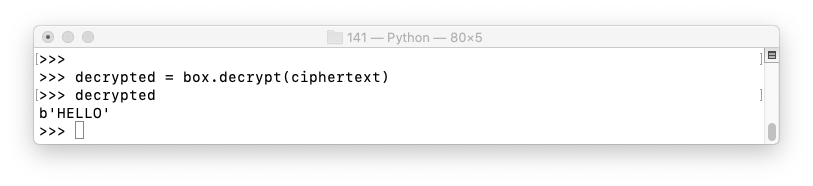## Using Base64

It's inconvenient to handle raw binary data like the ciphertext used above, so it's often converted to Base64.

Execute these commands to convert the ciphertext to Base64 and back.

```import base64 b64text = base64.b64encode(ciphertext).decode("ascii") b64text decoded = base64.b64decode(b64text) decoded ```
This produces printable Base64 text, and decoding it recovers the original binary ciphertext, as shown below.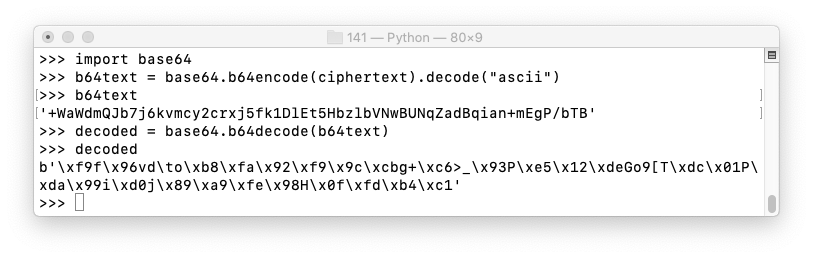# C 430.1: Decryption with the Key (5 pts)

This Python code specifies ciphertext:
```ciphertext = b'\x17\xbd\xc0\xa6\xeb\xff\\\x8e\x95T\x9b' ciphertext += b'\xd9\xe3\xa0\x06Tb?h\xc21@AYf\xe8\xce\xc0' ciphertext += b'\x83 \x10\xe8\xf2F\x00\xa9?l\x9f\x94/\xa9' ciphertext += b'\xbf\xb3\xabV\xe0\xef\xc4' ```
Decrypt it with this key to find the flag:
```key = b'11112222333344445555666677778888' ```

# C 430.2: Decryption with the Password (10 pts)

Decrypt this to find the flag:
• Salt: 1111222233334444
• Ciphertext (Base64-encoded): 9BIneuYE5L5tfYVHHLt+VV/r1kWc/kNVMRZdzW17+d5cl+251VilbMfWAErmYFDhidZJGW8=

# C 430.3: Guess the Password (20 pts)

Decrypt this to find the flag:
• Salt: abcdefghijklmnop
• The password is in this list: example.dict (alternate source: example.dict.zip)
• The password contains this string: kitten
• Ciphertext (Base64-encoded): hye3wygUWRQOiLrmqQNQZPKquKdwqdwSHDagK9cvUqT258w5kboSjXuzFgFFOft7TFcokN1a8Bk=

# C 430.4: Guess the Password (30 pts)

Decrypt this to find the flag:
• The salt is one digit repeated 16 times, like 5555555555555555
• The password is is from the same list as the previous challenge
• The password is more than 12 characters long
• The first character and the last character in the password are the same
• Ciphertext (Base64-encoded): KI8HpwBpIVaJaKE4sNuYBpxalojdQBHDJ6pLWcM4aTYJhgYdH7sQ5DhzXYx3sFxjg4BCAunFziUy

## Sources

How to securely encrypt data in Python with NaCl high level library
libsodium

Posted 11-13-20